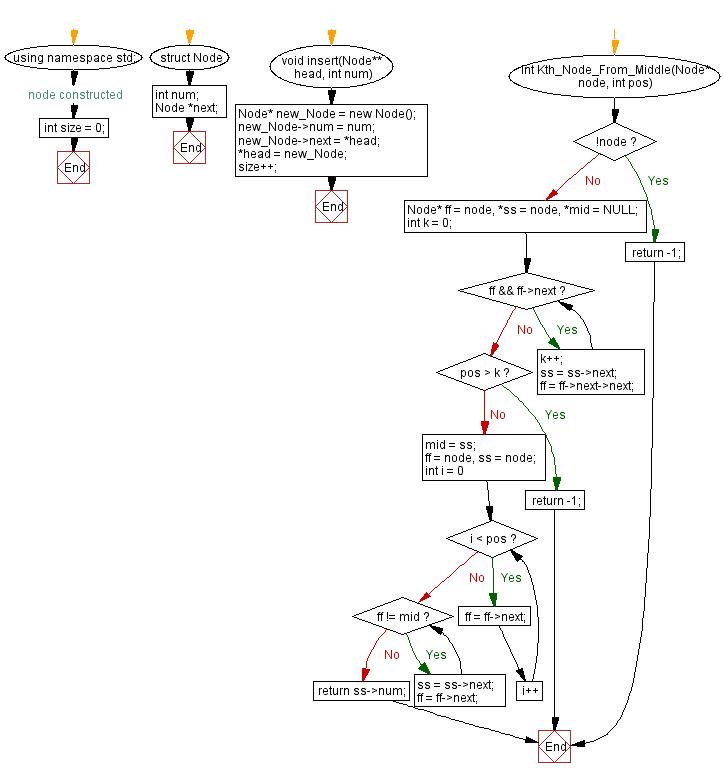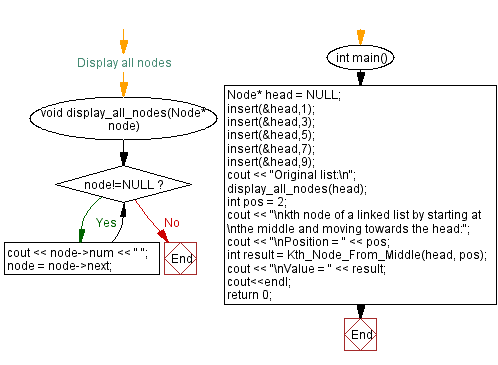﻿ C++ - Kth node from the Middle towards Head of a Linked List

## C++ Linked List: Exercise-14 with Solution

Write a C++ program to find the kth node of a linked list by starting at the middle and moving towards the head.

Test Data:
Original list:
9 7 5 3 1
kth node of a linked list by starting at
the middle and moving towards the head:
Position = 2
Value = 9

Sample Solution:

C++ Code:

``````#include <iostream>

using namespace std;

struct  Node
{
int num;
Node *next;
}; //node constructed

int size = 0;
Node* new_Node = new Node();
new_Node->num = num;
size++;
}

int Kth_Node_From_Middle(Node* node, int pos) {
if(!node)
return -1;
Node* ff = node, *ss = node, *mid = NULL;
int k = 0;
while(ff && ff->next)
{
k++;
ss = ss->next;
ff = ff->next->next;
}
if(pos > k)
return -1;
mid = ss;
ff = node, ss = node;
for(int i = 0; i < pos; i++)
{
ff = ff->next;
}
while(ff != mid)
{
ss = ss->next;
ff = ff->next;
}
return ss->num;
}

//Display all nodes
void display_all_nodes(Node* node)
{
while(node!=NULL){
cout << node->num << " ";
node = node->next;
}
}

int main()
{
cout << "Original list:\n";
int pos = 2;
cout << "\nkth node of a linked list by starting at \nthe middle and moving towards the head:";
cout << "\nPosition = " << pos;
cout << "\nValue = " << result;
cout<<endl;
return 0;
}
``````

Sample Output:

```Original list:
9 7 5 3 1
kth node of a linked list by starting at
the middle and moving towards the head:
Position = 2
Value = 9
```

Flowchart:CPP Code Editor: